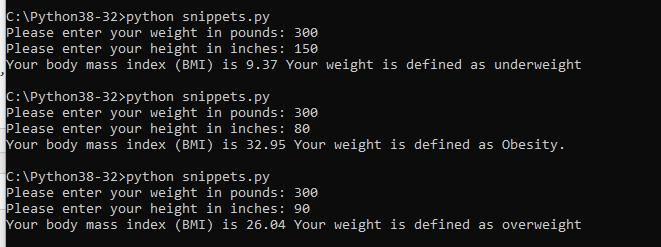Home » Body Mass Index calculator in Python

# Body Mass Index calculator in Python

Body mass index (BMI) is a value derived from the mass (weight) and height of a person. The BMI is defined as the body mass divided by the square of the body height, and is universally expressed in units of kg/m2, resulting from mass in kilograms and height in metres.

The BMI is a convenient rule of thumb used to broadly categorize a person as underweight, normal weight, overweight, or obese based on tissue mass (muscle, fat, and bone) and height.

There is some debate about whether the BMI scale is still accurate enough – you can read about the limitations at https://en.wikipedia.org/wiki/Body_mass_index#Limitations as well as reading about the history, formula and alternaticves but for a bit of fun its a nice little beginner friendly script to write in python

### Code

```#BMI Categories:
#Underweight = &lt;18.5
#Normal weight = 18.5–24.9
#Overweight = 25–29.9
#Obesity = BMI of 30 or greater

BMI = weight * 703 / (height ** 2)

if BMI < 18.5:
print('Your body mass index (BMI) is', format(BMI, '.2f'), 'Your weight is defined as underweight')
elif BMI > 18.5 and BMI < 25:
print('Your body mass index (BMI) is', format(BMI, '.2f'), 'Your weight is defined as normal')
elif BMI > 25 and BMI < 30:
print('Your body mass index (BMI) is', format(BMI, '.2f'), 'Your weight is defined as overweight')
else:
print('Your body mass index (BMI) is', format(BMI, '.2f'), 'Your weight is defined as Obesity.')```

### Overview

This is not that difficult example but for beginners here is a brief walkthrough

The first 5 lines or so are easty to understand this is simply an explanation of the BMI categories

```#BMI Categories:
#Underweight = <18.5
#Normal weight = 18.5–24.9
#Overweight = 25–29.9
#Obesity = BMI of 30 or greater
```

We then get the weight and height by prompting the user for input, converting it to a float and storing it into a variable

```weight = float(input('Please enter your weight in pounds: '))
```

The next line uses the formula to work out the BMI value for the user

```BMI = weight * 703 / (height ** 2)
```

We then have to display a message depending on the BMI value. The first one is easy as basically anything under 18.5 is classified as underweight

The next 2 are interesting as we need to display a line of text depending on whether the value is greater than or less than the value we also need to use the and logical operator as when the operands are true then the condition becomes true.

After all the conditions have been checked it can only be the else condition that is true

```if BMI < 18.5:
print('Your body mass index (BMI) is', format(BMI, '.2f'), 'Your weight is defined as underweight')
elif BMI > 18.5 and BMI < 25:
print('Your body mass index (BMI) is', format(BMI, '.2f'), 'Your weight is defined as normal')
elif BMI > 25 and BMI < 30:
print('Your body mass index (BMI) is', format(BMI, '.2f'), 'Your weight is defined as overweight')
else:
print('Your body mass index (BMI) is', format(BMI, '.2f'), 'Your weight is defined as Obesity.')
```

### Running It

Save this as bmi.py open a command prompt and run it using python bmi.py, here you can see me testing out some random databmi output

0 comment

#### You may also like

This website uses cookies to improve your experience. We'll assume you're ok with this, but you can opt-out if you wish. Accept Read More